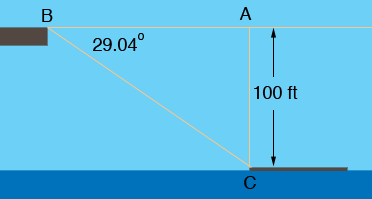SEARCH HOMEMath Central Quandaries & QueriesQuestion from joyce, a student: hello, here is my problem...... As you stand on a bridge w/c is 100 ft. above the water you are looking @ an approaching barge. If the A of top of the front of the bridge is 29.04 degrees and the angle of depression of the rear is 17.36 degrees . Find the length of the barge? Thank you in advance.....Hi Joyce,

You need to assume that the surface of the barge is on the surface of the water so that you are 100 feet above the barge.In the sketch above B is the bridge surface and C is the front end of the barge. The angle of depression is 29.04 degrees and hence

tan(29.04) = |CA|/|AB| = 100/|AB|

Solve for |AB|

In a similar fashion you can find the horizontal distance to the rear of the barge and hence determine the length of the barge.

HarleyMath Central is supported by the University of Regina and The Pacific Institute for the Mathematical Sciences.